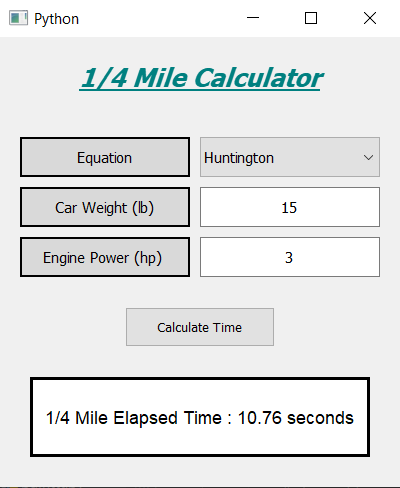Open in App
Not now

# 1/4 Mile Calculator using PyQt5 in Python

• Last Updated : 01 Aug, 2020

Prerequisite : Introduction to pyqt-5

In this article, we will see how we can create a quarter(1/4) mile calculator using PyQt5. Quarter mile calculator is used to determine the terminal speed (trap speed) and quarter-mile elapsed time (ET) of a vehicle based on its weight and power.

Below image shows how the 1/4 mile calculator looks like :1/4 mile calculator

The quarter-mile calculator employs three distinct empirical equations to determine the quarter-mile elapsed time (ET). These are as follows:

1. Roger Huntington’s Equation:

`Time = 6.290 × (Weight / Power)^(1/3)`

2. Geoffrey Fox’s Equations:

`Time = 6.269 × (Weight / Power)^(1/3)`

3. Patrick Hale’s Equations:

`Time = 5.825 × (Weight / Power)^(1/3)`

Note: Here weight is in Pound (lb) and power is in horsepower (hp) units.

GUI Implementation Steps :
1. Create a heading label that display the calculator name
2. Create label and combo box pair for selecting equation, label to show what user has to select and combo box to select
3. Similarly create a pair of label and line edit to get the weight and power of car
4. Create a push button to calculate the time
5. Create a label to show the calculated time

Back-End Implementation :
1. Make the combo box non editable
2. Make the line edit to accept only the number as input
3. Add action to the push button
4. Inside the push button action get the text of the line edits
5. Check if the line edit text is empty or zero then return so that function will not execute further
6. Convert the text value into integer
7. Get the selected option from the combo box and set constant value according to it
8. Calculate the time and show this value through the label

Below is the implementation :

## Python3

 `# importing required libraries``from` `PyQt5.QtWidgets ``import` `*``from` `PyQt5 ``import` `QtCore, QtGui``from` `PyQt5.QtGui ``import` `*``from` `PyQt5.QtCore ``import` `*`` ` `import` `sys`` ` ` ` `class` `Window(QMainWindow):`` ` `    ``def` `__init__(``self``):``        ``super``().__init__()`` ` `        ``# setting title``        ``self``.setWindowTitle(``"Python "``)`` ` `        ``# width of window``        ``self``.w_width ``=` `400`` ` `        ``# height of window``        ``self``.w_height ``=` `450`` ` `        ``# setting geometry``        ``self``.setGeometry(``100``, ``100``, ``                         ``self``.w_width, ``                         ``self``.w_height)`` ` `        ``# calling method``        ``self``.UiComponents()`` ` `        ``# showing all the widgets``        ``self``.show()`` ` `    ``# method for components``    ``def` `UiComponents(``self``):`` ` `        ``# creating head label``        ``head ``=` `QLabel(``"1/4 Mile Calculator"``, ``                      ``self``)`` ` `        ``# setting geometry to the head``        ``head.setGeometry(``0``, ``10``, ``400``, ``60``)`` ` `        ``# setting font``        ``font ``=` `QFont(``'Times'``, ``15``)``        ``font.setBold(``True``)``        ``font.setItalic(``True``)``        ``font.setUnderline(``True``)`` ` `        ``# setting font to the head``        ``head.setFont(font)`` ` `        ``# setting alignment of the head``        ``head.setAlignment(Qt.AlignCenter)`` ` `        ``# setting color effect to the head``        ``color ``=` `QGraphicsColorizeEffect(``self``)``        ``color.setColor(Qt.darkCyan)``        ``head.setGraphicsEffect(color)`` ` `        ``# creating a label``        ``e_label ``=` `QLabel(``"Equation"``, ``self``)`` ` `        ``# setting properties  label``        ``e_label.setAlignment(Qt.AlignCenter)``        ``e_label.setGeometry(``20``, ``100``, ``170``, ``40``)``        ``e_label.setStyleSheet(``"QLabel"``                              ``"{"``                              ``"border : 2px solid black;"``                              ``"background : rgba(70, 70, 70, 35);"``                              ``"}"``)``        ``e_label.setFont(QFont(``'Times'``, ``9``))`` ` `        ``# creating a combo box to selected equation``        ``self``.equation ``=` `QComboBox(``self``)`` ` `        ``# adding items to the combo box``        ``items ``=` `[``"Huntington"``, ``"Fox"``, ``"Hale"``]``        ``self``.equation.addItems(items)`` ` `        ``# setting properties to the combo box``        ``self``.equation.setFont(QFont(``'Times'``, ``9``))``        ``self``.equation.setGeometry(``200``, ``100``, ``180``, ``40``)``        ``self``.equation.setEditable(``False``)`` ` ` ` `        ``# creating a label``        ``w_label ``=` `QLabel(``"Car Weight (lb)"``, ``self``)`` ` `        ``# setting properties to the label``        ``w_label.setAlignment(Qt.AlignCenter)``        ``w_label.setGeometry(``20``, ``150``, ``170``, ``40``)``        ``w_label.setStyleSheet(``"QLabel"``                              ``"{"``                              ``"border : 2px solid black;"``                              ``"background : rgba(70, 70, 70, 35);"``                              ``"}"``)``        ``w_label.setFont(QFont(``'Times'``, ``9``))`` ` `        ``# creating a QLineEdit object``        ``self``.weight ``=` `QLineEdit(``self``)`` ` `        ``# accepting only number as input``        ``onlyInt ``=` `QIntValidator()``        ``self``.weight.setValidator(onlyInt)`` ` `        ``# setting properties to the line edit``        ``self``.weight.setGeometry(``200``, ``150``, ``180``, ``40``)``        ``self``.weight.setAlignment(Qt.AlignCenter)``        ``self``.weight.setFont(QFont(``'Times'``, ``9``))`` ` `        ``# creating a label``        ``p_label ``=` `QLabel(``"Engine Power (hp) "``, ``self``)`` ` `        ``# setting properties to the years label``        ``p_label.setAlignment(Qt.AlignCenter)``        ``p_label.setGeometry(``20``, ``200``, ``170``, ``40``)``        ``p_label.setStyleSheet(``"QLabel"``                              ``"{"``                              ``"border : 2px solid black;"``                              ``"background : rgba(70, 70, 70, 35);"``                              ``"}"``)``        ``p_label.setFont(QFont(``'Times'``, ``9``))`` ` `        ``# creating a QLineEdit object``        ``self``.power ``=` `QLineEdit(``self``)`` ` `        ``# accepting only number as input``        ``onlyInt ``=` `QIntValidator()``        ``self``.power.setValidator(onlyInt)`` ` `        ``# setting properties to the line edit``        ``self``.power.setGeometry(``200``, ``200``, ``180``, ``40``)``        ``self``.power.setAlignment(Qt.AlignCenter)``        ``self``.power.setFont(QFont(``'Times'``, ``9``))`` ` ` ` `        ``# creating a push button``        ``calculate ``=` `QPushButton(``"Calculate Time"``, ``self``)`` ` `        ``# setting geometry to the push button``        ``calculate.setGeometry(``125``, ``270``, ``150``, ``40``)`` ` `        ``# adding action to the calculate button``        ``calculate.clicked.connect(``self``.calculate_action)`` ` `        ``# creating a label to show percentile``        ``self``.result ``=` `QLabel(``self``)`` ` `        ``# setting properties to result label``        ``self``.result.setAlignment(Qt.AlignCenter)``        ``self``.result.setGeometry(``30``, ``340``, ``340``, ``80``)``        ``self``.result.setWordWrap(``True``)``        ``self``.result.setStyleSheet(``"QLabel"``                                  ``"{"``                                  ``"border : 3px solid black;"``                                  ``"background : white;"``                                  ``"}"``)``        ``self``.result.setFont(QFont(``'Arial'``, ``11``))`` ` `    ``# method for calculating the ``    ``# quarter-mile elapsed time (ET)``    ``def` `calculate_action(``self``):`` ` ` ` `        ``# getting weight of car``        ``weight ``=` `self``.weight.text()`` ` `        ``# getting power of engine``        ``power ``=` `self``.power.text()`` ` `        ``# if no input is given close the function``        ``if` `len``(weight) ``=``=` `0` `or` `len``(power) ``=``=` `0``:``            ``return`` ` `        ``# converting weight into integer``        ``weight ``=` `int``(weight)`` ` `        ``# converting power into integer``        ``power ``=` `int``(power)`` ` `        ``# if user enter total value as 0``        ``# return the function``        ``if` `power ``=``=` `0` `or` `weight ``=``=` `0``:``            ``return`` ` `        ``# getting the selected equation index``        ``equation ``=` `self``.equation.currentIndex()`` ` `        ``# setting constant value according to the equation``        ``# Roger Huntington's constant``        ``if` `equation ``=``=` `0``:``            ``constant ``=` `6.290`` ` `        ``# Geoffrey Fox's constant``        ``elif` `equation ``=``=` `1``:``            ``constant ``=` `6.269`` ` `        ``# Patrick Hale's constant``        ``else``:``            ``constant ``=` `5.825`` ` `        ``# calculating the time``        ``result ``=` `constant ``*` `((weight``/``power)``*``*``(``1``/``3``))`` ` `        ``# formatting the time``        ``result ``=` `"{:.2f}"``.``format``(result)`` ` `        ``# setting text to the result label``        ``self``.result.setText(``"1/4 Mile Elapsed Time : "``                            ``+` `str``(result) ``+` `" seconds"``)`` ` ` ` `# create pyqt5 app``App ``=` `QApplication(sys.argv)`` ` `# create the instance of our Window``window ``=` `Window()`` ` `# start the app``sys.exit(App.``exec``())`

Output :

My Personal Notes arrow_drop_up